All submissions of the EM system will be redirected to Online Manuscript Submission System. Authors are requested to submit articles directly to Online Manuscript Submission System of respective journal.

# Original Article

, Volume: 5( 4)

## Relationships Between Two Gravitationally-Bound Points in Single or Multiple Systems In The Universe

*Correspondence:
Jihai Zhang Sr.Electrinics Designer, Canada, Tel: 613-721-2937; E-mail: [email protected]

Received Date: June 29, 2017 Accepted Date: July 20, 2017 Published Date: August 06, 2017

Citation: Zhang J and Tao P2. Relationships Between Two Gravitationally-Bound Points in Single or Multiple Systems In The Universe. J Phys Astron. 2017;5(2):116.

### Abstract

Supported by real data, this article derives and proves relationships for any two gravitationally-bound objects in single or multiple systems in the universe. These findings have implications for simpler and more accurate calculations in related practical applications . Normally, relative error is less than 3.35%.

### Keywords

Gravity; Centrifugul force; N body; Gravitational field; Solar system; Exoplanet; Universe

### Introduction

Gravitationally-bound objects that orbit a central object (single system) or different central objects (multiple systems) are general phenomena in the universe. It is therefore very meaningful to find the relationships between two gravitationally-bound points in single or multiple systems in the universe.

In this article, the case of the common centre is first discussed (single system examples: planets orbiting the Sun, moons orbiting a planet) and then, the second case of multiple centres with different central masses is discussed (multiple system examples: different moons orbiting different planets).

In these two systems, results show that the relationship between gravity and centrifugal force is the same, however, the relationships between any two gravitationally-bound points are different but convertible, and normally, the maximum relative error is less than 3.35%.

### Derivation and Verification

Relationships between two points around the same centre (single system):

Centrifugal force:(1)

then(1b)

Where, m is the mass of an orbiting body.

Gravitation:(2)

Then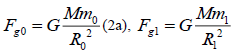(2b)

Where, M is the mass of the centre.(3)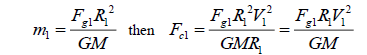(4)(5)

When(6)

Or(7)

Then(8)

Following are verifications of equation (7) :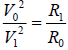using real data.

Verifications between the 8 planets in the Solar System as orbiting satellites and the Sun as the common centre.

Where absolute error(9)

Relative error(10)

Table 1, Table 2 and Table 3 show that E1max is less than 2.11%, due to the source data being averages. The results proved

equation (7):(8).

Planet V0
(km/s)
R0
(km)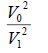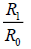Neptune 5.43 4495.06 0.033246832 0.03328098 3.41473E-05 0.001026557
Uranus 6.8 2872.46 0.052139689 0.052080795 5.88944E-05 0.001130189
Saturn 9.68 1433.53 0.105657743 0.104357774 0.001299968 0.012379737
Jupiter 13.06 778.57 0.192325543 0.192147142 0.000178401 0.000928031
Mars 24.07 227.92 0.653285161 0.656370656 0.003085495 0.004711918
Venus 35.02 108.21 1.382874908 1.382496997 0.000377791 0.000273317
Mercury 47.36 57.91 2.529146582 2.583318943 0.054172361 0.021192264

Table 1. Where V1 and R1 are Earth’s data, V1= 29.78 (km/s), R1=149598023 (km). Note: V1 and R1 can be data from any of the 8 planets (verified and confirmed) .

Moon of Jupiter V0
(km/s)
R0
(km)Europa  13.74 670900 0.628312762 0.628558652 0.000245891 0.000391274
Ganymede  10.88 1070400 0.393967327 0.393964872 2.45406E-06 6.2291E-06
Callisto  8.204 1882700 0.22400294 0.223986827 1.61126E-05 7.19328E-05

Table 2. Verifications between Jupiter’s 4 moons. Where V1 and R1 are moon Io’s data, V1= 17.334 (km/s) , R1=421700 (km) . Note: V1 and R1 can be data from any of the 4 moons (verified and confirmed).

Moon of Saturn V0
(km/s)
R0
(km)Tethys  11.35 294619 0.631735537 0.629759112 0.001976273 0.003133465
Rhea  8.48 527108 0.352643018 0.351994278 0.00064874 0.001841344
Titan  5.57 1221870 0.1521437 0.151848396 0.000295304 0.001942842

Table 3. Verifications between Saturn’s 4 moons. Where V1 and R1 are moon Mimas’ data, V1= 14.28 (km/s) , R1=185539 (km) . Note: V1 and R1 can be data from any of the 4 moons (verified and confirmed).

Relationship Between Two Points Around the Different Centres (Multiple Systems):

There are two kinds of relationships in a multiple system, one is the relationship between any two points around the same centre (single system) and another is the relationship between any two points around different centres (multiple systems). The following discusses relationships found in multiple systems, however the formerly discussed single system relationships are still valid and applied [4-10].

According to equation (3),(11)

Where M0 is the central mass of single system A, R0 is the distance from the orbiting point 0 to the centre.

According to equation (4),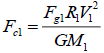(12)

Where M1 is the central mass of single system B, R1 is the distance from the orbiting point 1 to the centre.

Then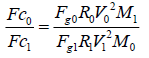(13)

When(14)

Then(15)

When(16)

Then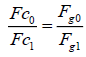(17)

Following are Verifications Of Equation (16) :Using Real Data( V0 And R0 are Theoretical Data).

Example of detailed calculations between Jupiter’s moon Io and Saturn’s moon Mimas:

Where M1, V1 and R1 are Jupiter’s data and M0, V0 and Vx and Rx are Saturn’s data, V0 and R0 are Saturn’s theoretical data obtained from real data.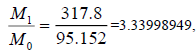R1=421700(km)(moon Io), according to equation( 16):Vx=14.28(km/s), Rx=85539(km)( moon Mimas), according to equation( 7):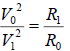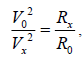thenTable 4 shows that E1max is 0.00488. The results proved equation (16):and therefore, equation (8):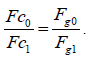Moons M0 Vx (km/s) Rx (km) R0 (k [m) V0 (km/s)Mimas  95.152  14.28 185539 1408473.568 5.18288 3.34 3.344 0.00134
Miranda   14.536  6.66 129390 9219610.622 0.78898 21.86 21.97 0.00488
Phobos  0.107  2.138 9376 1252488411 0.00585 2970 2963 0.00231
Moon  1  1.022 384399 134016260 0.05473 317.8 316.7 0.0035

Table 4. Verifications between Jupiter’s moon Io, Saturn’s moon Mimas, Uranus’ moon Miranda, Mars’ moon Phobos and Earth’s moon. These moons orbit different centres with different central masses (this multiple system consists of 5 single systems). Note: Although M1, V1 and R1 are Jupiter’s data (M1=317.8 , V1=17.334 (km/s)  and R1=421700 (km) ), these data can be from any of the 5 single systems (verified and confirmed).

### Conclusion(8)

Where, Fc0 and Fc1 are centrifugal forces of any two points around the same or different centres, Fg0 and Fg1 are gravitational forces with distances of R0 and R1 to the corresponding centres.

Relationship between two points around the same centre (single system):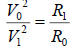(7

Where, V0 and V1 are cross-radial velocities with distances of R0 and R1 to the centre respectively [Tables 5-7].

Moons M0 V0(km/s) R0(km) k Average k
Mimas 95.152 14.28 185539 2.51493E-06 2.50863E-06
Miranda 14.536 6.66 129390 2.53277E-06
Phobos 0.107 2.138 9376 2.49661E-06
Moon 1 1.022 384399 2.49067E-06
Io 317.8 17.334 421700 2.50815E-06

Table. 5. calculations of constant k based on the data of the 5 planets and their moons

Planet   V0(km/s) R0(k[m) k MSUN E1
Neptune 5.43 4.50E+09 2.51E-06 3.32E+05 -0.001547221
Uranus 6.81 2.87E+09 2.51E-06 3.34E+05 0.003553643
Saturn 9.69 1.43E+09 2.51E-06 3.38E+05 0.014020461
Jupiter 13.07 7.79E+08 2.51E-06 3.34E+05 0.001938855
Mars 24.13 2.28E+08 2.51E-06 3.33E+05 -0.0002545
Venus 35.02 1.08E+08 2.51E-06 3.33E+05 -0.000248513
Mercury 47.87 5.79E+07 2.51E-06 3.33E+05 -0.000292851
Earth 29.78 149598023 2.51E-06 3.33E+05 -0.000534934

Table. 6. verification results of equation (17):to calculate the mass of the Sum and error E1 based on the data of the 8 planets and the Sun(M=333000) to confirm k=2.50863E-6.

Exoplanet R(AU) T(day) M M1 E1
Centauri 0.0485 11.186 0.12121 0.121612946 0.003318847
PSR B1257 0.19 25.262 1.4 1.433605191 0.023719035
 0.36 66.5419 1.4 1.405469195 0.003898952
0.46 98.2114 1.4 1.346027444 -0.039309553
Gliese436 0.0291 2.6339 0.46 0.473788621 0.029532638

Table. 7. verification results of equation (17) using exoplanet data( R, T and M), M is real central star mass, M1 is calculation central star mass, E1 is Error( max: 3.9%, average: 1.28% ).

Relationship between two points around the different centres (multiple systems):(16)

Where, M0 and M1 are corresponding central masses, V0 and V1 are cross-radial velocities with distances of R0 and R1 to the centres respectively.

Gravitationally-bound point in single or multiple systems in the universe:

According to (15):(17)

whereunit of M1 is Earth mass

Where, V0 and V1 are cross-radial velocities with distances of R0 and R0 to the centre respectively.

### Explanation

This article finds and establishes the theoretical/ideal relationships between two gravitationally-bound points in single or multiple systems in the universe, and uses real data to verify the calculation errors (the real statistic impact of the N body issue, N = 9, 8 planets + Sun) in these relationships. Especially, Equations (7),(16) and (17) are independence in gravity and centrifugal force, (17) is verified and confirmed by real data from both local and exoplanets.

Normally, when the orbit of a gravitationally-bound point approximates a circle, the theoretical/ideal relationships between two gravitationally-bound points can be expressed by equations (7), (8),(16) and (17) with about 3.35% error ( average: 0.6%). The N body issue exists, however, its impact is limited / even negligible.

Equation (8) represents the relationship between gravity and centrifugal force. According to equation (8), gravity and centrifugal force change in the same direction at the same ratio [11-22].

Equation (17) represents the relationship between gravitationally-bound point and central mass. According to equation (17), once we know any two of the factors: cross-radial velocity V1, the central mass M1 or the distance R1, we can calculate the corresponding distance R1 or central mass M1 or cross-radial velocity V1 of a moon, a planet or a star.

Given that k is an universal constant. Now we can use equations (17) to explain why there are many exoplanets orbit their central stars at very high speed V in a very short distance R.

In summary, these findings are properties of gravitational field, prove physical laws are universal and can be used to accurately identify exoplanets.

### References

Welcome Message

Select your language of interest to view the total content in your interested language

###### Citations : 260

Journal of Physics & Astronomy received 260 citations as per Google Scholar report

#### Indexed In

• Open J Gate
• China National Knowledge Infrastructure (CNKI)
• Cosmos IF
• Directory of Research Journal Indexing (DRJI)
• MIAR
• Secret Search Engine Labs
• Euro Pub
• clarivate-web-of-science-logo-vector

### 9th International Conference on Physics

Edinburgh, Scotland

### 6th International Conference on Astronomy, Astrophysics and Space Science

Budapest, Hungary

Berlin, Germany

### 9th International Conference on Physics

Edinburgh, Scotland

### 6th International Conference on Astronomy, Astrophysics and Space Science

Budapest, Hungary

### World Congress on Quantum Physics

Berlin, Germany

#### For Librarians#### Open Access Journals